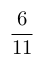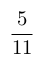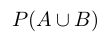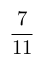Select Page

# MCQ in English Solutions for CBSE Maths 12 Science Matrices

MCQ in English Solutions for CBSE Maths 12 Science Matrices to enable students to get Solutions in a narrative video format for the specific question.

Expert Teacher provides MCQ Solutions for CBSE Maths 12 Science Matrices through Video Solutions in English language. This video solution will be useful for students to understand how to write an answer in exam in order to score more marks. This teacher uses a narrative style for a question from Matrices not only to explain the proper method of answering question, but deriving right answer too.

Please find the question below and view the Solution in a narrative video format.

Question:

Solution Video in English:

You can select video Solutions from other languages also. Please check Solutions in ( Hindi )

## Similar Questions from CBSE, 12th Science, Maths, Matrices

Question 1 : Find the transpose of the matrix:. (View Answer Video)

Question 2 : LetFind AB. (View Answer Video)

Question 3 : Find the value of x, if. (View Answer Video)

Question 4 : Given,, find the value of z. (View Answer Video)

Question 5 : Compute:. (View Answer Video)

### Continuity and Differentiability

Question 1 : Differentiate the function w.r.t.x. (View Answer Video)

Question 2 : Differentiate the functionwith respect to x. (View Answer Video)

Question 3 : Ifand, find. (View Answer Video)

Question 4 : Findfor the function. (View Answer Video)

Question 5 : Findfor the function. (View Answer Video)

### Integrals

Question 1 : Evaluate :(View Answer Video)

Question 2 : Evaluate :(View Answer Video)

Question 3 : Find. (View Answer Video)

Question 4 : Find the integral of the function. (View Answer Video)

Question 5 : Evaluate :(View Answer Video)

### Probability

Question 1 : A die is thrown 6 times. If 'getting an odd number' is a 'success', what is the probability of obtaining:
atleast 5 successes?, Find P(not E and not F). (View Answer Video)
Question 4 : If P(F) = 0.35 and=0.85 and E and F are independent events. Find P(E).  (View Answer Video)
Question 5 : If P(A)=, P(B)=and=, find. (View Answer Video)